# 2-D IFFT

2-D Inverse fast Fourier transform of input

## Library

Transforms

`visiontransforms`

•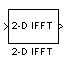## Description

The 2-D IFFT block computes the inverse fast Fourier transform (IFFT) of an M-by-N input matrix in two steps. First, it computes the one-dimensional IFFT along one dimension (row or column). Next, it computes the IFFT of the output of the first step along the other dimension (column or row).

The output of the IFFT block is equivalent to the MATLAB® `ifft2` function:

```y = ifft2(A) % Equivalent MATLAB code ```

Computing the IFFT of each dimension of the input matrix is equivalent to calculating the two-dimensional inverse discrete Fourier transform (IDFT), which is defined by the following equation:

`$f\left(x,y\right)=\frac{1}{MN}\sum _{m=0}^{M-1}\sum _{n=0}^{N-1}F\left(m,n\right){e}^{j\frac{2\pi mx}{M}}{e}^{j\frac{2\pi ny}{N}}$`

where $0\le x\le M-1$ and $0\le y\le N-1$.

The output of this block has the same dimensions as the input. If the input signal has a floating-point data type, the data type of the output signal uses the same floating-point data type. Otherwise, the output can be any fixed-point data type. The block computes scaled and unscaled versions of the IFFT.

The input to this block can be floating-point or fixed-point, real or complex, and conjugate symmetric. The block uses one of two possible FFT implementations. You can select an implementation based on the FFTW library , , or an implementation based on a collection of Radix-2 algorithms. You can select `Auto` to allow the block to choose the implementation.

### Port Description

PortDescriptionSupported Data TypesComplex Values Supported

Input

Vector or matrix of intensity values

• Double-precision floating point

• Single-precision floating point

• Fixed point

• 8-, 16-, 32-bit signed integer

• 8-, 16-, 32-bit unsigned integer

Yes

Output

2-D IFFT of the input

Same as Input port

Yes

### FFTW Implementation

The FFTW implementation provides an optimized FFT calculation including support for power-of-two and non-power-of-two transform lengths in both simulation and code generation. Generated code using the FFTW implementation will be restricted to MATLAB host computers. The data type must be floating-point. Refer to Simulink Coder for more details on generating code.

The Radix-2 implementation supports bit-reversed processing, fixed or floating-point data, and allows the block to provide portable C-code generation using the Simulink Coder. The dimensions of the input matrix, M and N, must be powers of two. To work with other input sizes, use the Image Pad block to pad or truncate these dimensions to powers of two, or if possible choose the FFTW implementation.

With Radix-2 selected, the block implements one or more of the following algorithms:

• Butterfly operation

• Double-signal algorithm

• Half-length algorithm

Radix-2 Algorithms for Real or Complex Input Complexity Floating-Point Signals

Parameter Settings

Algorithms Used for IFFT Computation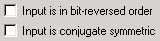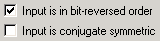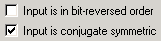Butterfly operation and radix-2 DIT in conjunction with the half-length and double-signal algorithms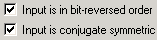Radix-2 DIF in conjunction with the half-length and double-signal algorithms

Radix-2 Algorithms for Real or Complex Input Complexity Fixed-Point Signals

Other Parameter Settings

Algorithms Used for IFFT ComputationNote

The Input is conjugate symmetric parameter cannot be used for fixed-point signals.

Radix-2 Optimization for the Table of Trigonometric Values

In certain situations, the block’s Radix–2 algorithm computes all the possible trigonometric values of the twiddle factor

`${e}^{j\frac{2\pi k}{K}}$`

where K is the greater value of either M or N and $k=0,\cdots ,K-1$. The block stores these values in a table and retrieves them during simulation. The number of table entries for fixed-point and floating-point is summarized in the following table:

Number of Table Entries for N-Point FFT

floating-point

3 N/4

fixed-point

N

Fixed-Point Data Types

The following diagrams show the data types used in the IFFT block for fixed-point signals. You can set the sine table, accumulator, product output, and output data types displayed in the diagrams in the IFFT dialog box as discussed in Parameters.

Inputs to the IFFT block are first cast to the output data type and stored in the output buffer. Each butterfly stage then processes signals in the accumulator data type, with the final output of the butterfly being cast back into the output data type. The block multiplies in a twiddle factor before each butterfly stage in a decimation-in-time IFFT and after each butterfly stage in a decimation-in-frequency IFFT.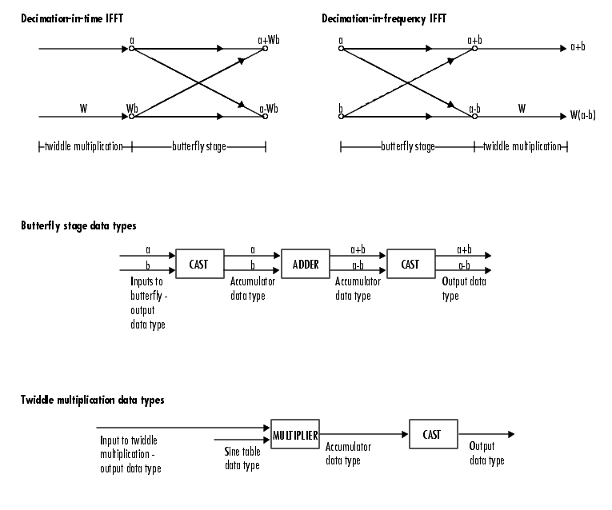The multiplier output appears in the accumulator data type because both of the inputs to the multiplier are complex. For details on the complex multiplication performed, refer to Multiplication Data Types.

## Parameters

FFT implementation

Set this parameter to `FFTW` ,  to support an arbitrary length input signal. The block restricts generated code with FFTW implementation to MATLAB host computers.

Set this parameter to `Radix-2` for bit-reversed processing, fixed or floating-point data, or for portable C-code generation using the Simulink Coder. The dimensions of the input matrix, M and N, must be powers of two. To work with other input sizes, use the Image Pad block to pad or truncate these dimensions to powers of two, or if possible choose the FFTW implementation. See Radix-2 Implementation.

Set this parameter to `Auto` to let the block choose the FFT implementation. For non-power-of-two transform lengths, the block restricts generated code to MATLAB host computers.

Input is in bit-reversed order

Select or clear this check box to designate the order of the input channel elements. Select this check box when the input should appear in reversed order, and clear it when the input should appear in linear order. The block yields invalid outputs when you do not set this parameter correctly. This check box only appears when you set the FFT implementation parameter to `Radix-2` or `Auto`.

For more information ordering of the output, see Bit-Reversed Order. The 2-D FFT block bit-reverses the order of both the columns and the rows.

Input is conjugate symmetric

Select this option when the block inputs both floating point and conjugate symmetric, and you want real-valued outputs. This parameter cannot be used for fixed-point signals. Selecting this check box optimizes the block's computation method.

The FFT block yields conjugate symmetric output when you input real-valued data. Taking the IFFT of a conjugate symmetric input matrix produces real-valued output. Therefore, if the input to the block is both floating point and conjugate symmetric, and you select this check box, the block produces real-valued outputs.

If the IFFT block inputs conjugate symmetric data and you do not select this check box, the IFFT block outputs a complex-valued signal with small imaginary parts. The block outputs invalid data if you select this option with non conjugate symmetric input data.

Divide output by product of FFT length in each input dimension

Select this check box to compute the scaled IFFT. The block computes scaled and unscaled versions of the IFFT. If you select this option, the block computes the scaled version of the IFFT. The unscaled IFFT is defined by the following equation:

`$f\left(x,y\right)=\sum _{m=0}^{M-1}\sum _{n=0}^{N-1}F\left(m,n\right){e}^{j\frac{2\pi mx}{M}}{e}^{j\frac{2\pi ny}{N}}$`

where $0\le x\le M-1$ and $0\le y\le N-1$.

The scaled version of the IFFT multiplies the above unscaled version by $\frac{1}{MN}$.

Rounding mode

Select the Rounding Modes for fixed-point operations. The sine table values do not obey this parameter; instead, they always round to `Nearest`.

Saturate on integer overflow

Select the overflow mode for fixed-point operations. See Precision and Range. The sine table values do not obey this parameter; instead, they are always saturated.

Sine table data type

Choose how you specify the word length of the values of the sine table. The fraction length of the sine table values always equals the word length minus one. You can set this parameter to:

• A rule that inherits a data type, for example, ```Inherit: Same word length as input```

• An expression that evaluates to a valid data type, for example, `fixdt(1,16)`

The sine table values do not obey the Rounding mode and Saturate on integer overflow parameters; instead, they are always saturated and rounded to `Nearest`.

Product output data type

Specify the product output data type. See Fixed-Point Data Types and Multiplication Data Types for illustrations depicting the use of the product output data type in this block. You can set this parameter to:

• A rule that inherits a data type, for example, ```Inherit: Inherit via internal rule```

• An expression that evaluates to a valid data type, for example, `fixdt(1,16,0)`

Click the buttonto display the Data Type Assistant, which helps you set the Product output data type parameter.

Accumulator data type

Specify the accumulator data type. SeeFixed-Point Data Types for illustrations depicting the use of the accumulator data type in this block. You can set this parameter to:

• A rule that inherits a data type, for example, ```Inherit: Inherit via internal rule```

• An expression that evaluates to a valid data type, for example, `fixdt(1,16,0)`

Click the buttonto display the Data Type Assistant, which helps you set the Accumulator data type parameter.

Output data type

Specify the output data type. See Fixed-Point Data Types for illustrations depicting the use of the output data type in this block. You can set this parameter to:

• A rule that inherits a data type, for example, ```Inherit: Inherit via internal rule```.

When you select `Inherit: Inherit via internal rule`, the block calculates the output word length and fraction length automatically. The internal rule first calculates an ideal output word length and fraction length using the following equations:

• When you select the Divide butterfly outputs by two check box, the ideal output word and fraction lengths are the same as the input word and fraction lengths.

• When you clear the Divide butterfly outputs by two check box, the block computes the ideal output word and fraction lengths according to the following equations:

`$W{L}_{idealoutput}=W{L}_{input}+floor\left({\mathrm{log}}_{2}\left(FFTlength-1\right)\right)+1$`
`$F{L}_{idealoutput}=F{L}_{input}$`

Using these ideal results, the internal rule then selects word lengths and fraction lengths that are appropriate for your hardware. For more information, see Inherit via Internal Rule.

• An expression that evaluates to a valid data type, for example, `fixdt(1,16,0)`

Click the buttonto display the Data Type Assistant, which helps you set the Output data type parameter.

Lock data type settings against change by the fixed-point tools

Select this parameter to prevent the fixed-point tools from overriding the data types you specify on the block mask. For more information, see `fxptdlg` (Fixed-Point Designer), a reference page on the Fixed-Point Tool in the Simulink® documentation.

## References

 Frigo, M. and S. G. Johnson, “FFTW: An Adaptive Software Architecture for the FFT,”Proceedings of the International Conference on Acoustics, Speech, and Signal Processing, Vol. 3, 1998, pp. 1381-1384.

 2-D DCT Computer Vision Toolbox™ software 2-D FFT Computer Vision Toolbox software 2-D IDCT Computer Vision Toolbox software 2-D FFT Computer Vision Toolbox software `bitrevorder` (Signal Processing Toolbox) Signal Processing Toolbox software `fft` MATLAB `ifft` MATLAB Simulink Coder Simulink

## Extended Capabilities

Introduced before R2006a